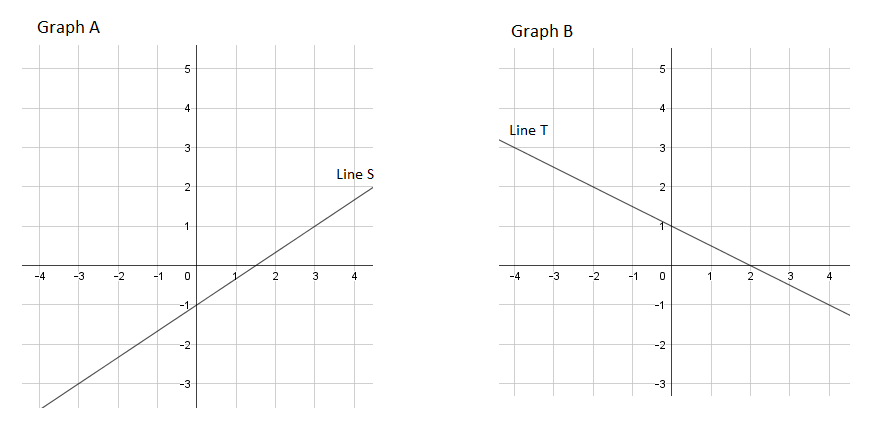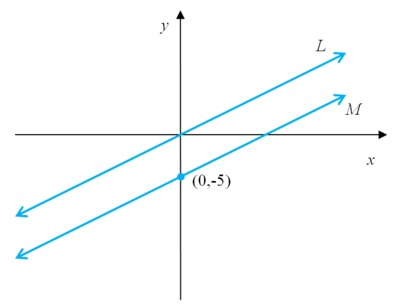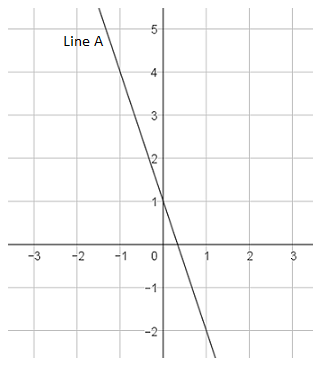# Linear Relationships

## Objective

Write linear equations for parallel and perpendicular lines.

## Common Core Standards

### Core Standards

?

• 8.EE.B.6 — Use similar triangles to explain why the slope m is the same between any two distinct points on a non-vertical line in the coordinate plane; derive the equation y = mx for a line through the origin and the equation y = mx + b for a line intercepting the vertical axis at b.

?

• 8.G.A.1

• 8.G.A.2

## Criteria for Success

?

1. Understand that parallel lines have the same slope.
2. Understand that perpendicular lines have opposite and reciprocal slopes.
3. Write equations for parallel and perpendicular lines using the relationship between slopes.

## Tips for Teachers

?

This lesson is a good opportunity to review and spiral in concepts from throughout the unit.

#### Remote Learning Guidance

If you need to adapt or shorten this lesson for remote learning, we suggest prioritizing Anchor Problem 1 (benefits from discussion) and Anchor Problem 2 (benefits from worked example). Find more guidance on adapting our math curriculum for remote learning here.

#### Fishtank Plus

• Problem Set
• Student Handout Editor
• Vocabulary Package

## Anchor Problems

?

### Problem 1

In Graph A, translate line S 3 units up to create parallel line S’.

• What is the slope of line S’?
• What is the relationship between the slopes of the two parallel lines?

In Graph B, rotate line T 90° clockwise to create perpendicular line T’.

• What is the slope of Line T?
• What is the slope of Line T’?
• What is the relationship between the slopes of the two perpendicular lines?### Problem 2

Lines L and M have the same slope. The equation of line L is ${4y=x}$
Line M passes through the point (0, -5).What is the equation of line M?

#### References

Illustrative Mathematics Proportional relationships, lines, and linear equations

Proportional relationships, lines, and linear equations, accessed on Feb. 24, 2018, 10:19 p.m., is licensed by Illustrative Mathematics under either the CC BY 4.0 or CC BY-NC-SA 4.0. For further information, contact Illustrative Mathematics.

### Problem 3

Line P passes through the points (0, 5) and (4, -3).

Line Q is perpendicular to line P and passes through the point (8, -2).

What is the equation for line Q?

## Problem Set

?The following resources include problems and activities aligned to the objective of the lesson that can be used to create your own problem set.

• Include spiraled problems from earlier in the unit, targeted at where students may need additional concept development or practice.
• Challenge: Another form of a linear equation is called point-slope form and is represented as $y-b=m(x-a)$, where $m$ is the slope and $(a, b)$ represents a point on the line. Write an equation of the line, in point-slope form, that passes through the points $(-2, 6)$ and $(3, -4)$
• Illustrative Mathematics Find the ChangeThis task reviews and helps to solidify concepts of slope and y-intercept; would make a good pair, collaborative problem.
• Kuta Software Free Algebra 2 Worksheets Review of Linear EquationsMany of these problems ask for standard form; change this to slope-intercept form.
• MARS Formative Assessment Lesson for Grade 8 Defining Lines by Points, Slopes and EquationsThis lesson pulls together concepts from the last few lessons.

?

Line A is shown in the graph.• Line B is parallel to line A and passes through the point (2, 3).
• Line C is perpendicular to line A and passes through the point (0, -4).

Write an equation for line B and for line C.

?